The RI8575A Phase Noise Discriminator TIM measures phase noise of RF signals, and provides supporting circuitry for characterization of RF sources.

Designed primarily for testing VCOs, it includes:
• Phase Noise measurement from 1 to 16 GHz.
• FM sideband measurement from 1 to 16 GHz.
• Phase detector with a built-in crystal reference oscillator, to lock the signal-under-test to the system timebase.
• Parametric supply/measure to supply and measure tune voltages, or tune currents.
• 16 bit frequency counter for linearity or Kv measurements.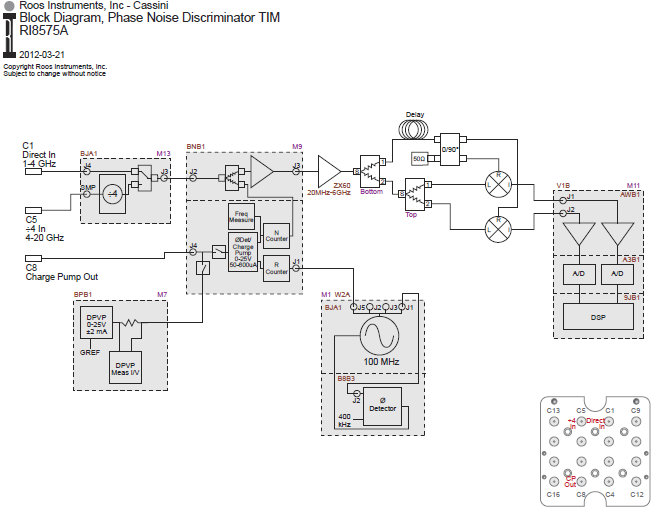Other Measurements

Other measurements accessed through a 'Pick Meas' function primarily for service, and not used for normal testing.

CONTROL SOFTWARE OPERATION:

The following is a description of the buttons used to control the RI8575A Phase Noise Discriminator TIM. This document is organized to loosely follow the order of the buttons in the RI8575A's editor panels.

Each panel can have 'State' buttons or 'Measurement' buttons. State buttons are used to set up an instrument. Measurement buttons perform a measurement, and return a result.

State Panel, State Buttons: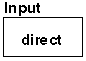The Input button defines which input is used, the direct or the /4 (divide-by-4) input.

Direct: Sets the TIM to measure at the Direct, 1-4 GHz input.

/4: Sets the TIM to measure at the divide-by-4 input.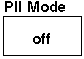The PLL Mode button defines what mode the TIM will use for measurements.

off: Turns off the RF path. This mode is useful when using the parametric measure for leakage measurements.

lock: Sets the TIM to drive the CP out from the phase detector, to phase lock the input source, while enabling the phase noise discriminator.
count: Sets the TIM to the frequency counter mode, to count the frequency of the input source.
disc: Discriminator-only mode, to measure phase noise without phase locking the DUT.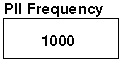The phase lock function uses a fractional divide. The frequency is controlled by the 'Pll Frequency', 'Pll Ref Freq', and 'Pll Ref Mod buttons'.

The Pll Frequency button is used to set the lock frequency in megahertz (MHz). Range is 800 to 4000 MHz for the direct input or 2000 to 16000 MHz for the /4 input.

'Pll Frequency' / 'Pll Ref Freq' sets the N Counter, with 'Pll Ref Mod' defining the allowable fractional part of the N Counter (and thus the resolution).

The resolution is set by the formula:
Direct Input: 'Pll Ref Freq' / 'Pll Ref Mod'
/4 Input: ('Pll Ref Freq' / 'Pll Ref Mod') * 4

Note that when 'Pll Frequency' / 'Pll Ref Frequency' results in a fraction, the phase lock will not be optimum due to the N Counter's fractional divide. For optimum phase lock performance, 'Pll Frequency' / 'Pll Ref Frequency' should be an integer.

When 'Pll Mode' is set to 'Count', 'Pll Frequency' and 'Pll Ref Freq' are used to set the N Counter, which then divides the input before the count circuitry. In 'Count' mode, 'PLL Frequency' should be set to the highest frequency expected.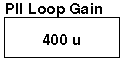The Pll Loop Gain button sets the phase detector current gain in amps. Max is 625u, min 10u. The loop gain is primarily a function of the external loop filter and the VCO's kV.

Programs such as Analog Devices' ADISimPll (https://form.analog.com/Form_Pages/RFComms/ADISimPll.aspx) can assist in determining the loop filter design and the Pll Loop Gain setting. The specific PLL referenced is the ADF4150HV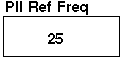The Pll Ref Freq button sets the reference frequency, in MHz, for the phase detector. This button controls the R Counter in the phase lock circuitry. 'Pll Ref Freq' is set by the formula 100 MHz/R, where R is an integer number from 4 to 1023. 25MHz is the maximum Pll Ref Freq.

When 'Pll Mode' is set to 'Count', 'Pll Frequency' and 'Pll Ref Freq' are used to set the N Counter. It is recommended that 'Pll Ref Freq' button be set to 25 MHz, when in count mode.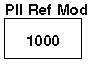The Pll Ref Mod button sets the allowable fractions for the fractional divide, thus setting the frequency resolution. Allowable values are from 1 to 4095. The frequency resolution is set by the formula:
Direct Input: 'Pll Ref Freq' / 'Pll Ref Mod'
/4 Input: ('Pll Ref Freq' / 'Pll Ref Mod') * 4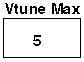The Vtune Max button sets the upper limit for the vTune drive voltage. The charge pump out will not exceed this voltage. Maximum settable limit is 25 volts. Minimum settable limit is 5 volts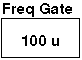The Freq Gate button sets the gate time, in seconds, for the frequency counter measurement. The frequency counter depth is 16 bits.

Resolution, in Hz, is:
(1/'Freq Gate') * ('Pll Frequency'/'Pll Ref Freq')

It is recommended that 'Pll Ref Freq' be set to 25 MHz, when in count mode.

Maximum 'Freq Gate' is: ('PllFrequency'/Fin) * (1/'Pll Ref Freq') * 65536
where Fin is the input frequency.

If Fin = 'PllFrequency' and 'Pll Ref Freq' is at the recommended 25 MHz, then:
Maximum 'Freq Gate' is: 2.6 mS

State Panel, Measurement Buttons: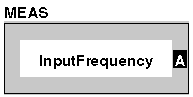The MEAS Frequency button performs a frequency measurement, in MHz, when 'Pll Mode' is set to 'Count'. For information on resolution and gate time, reference the 'Freq Gate' button. Note that the 'Pll Frequency' button should be set to the maximum expected frequency.

If 'Pll Mode' is set to 'Lock', then the parametric measure's 'Measure Mode' (from the Tune Measure panel) determines whether the MEAS Frequency button or the MEAS Lock button will function. If 'Measure Mode' is set to anything except 'None', then the MEAS Frequency button will function. This can be useful as a debug tool. If the Measure Mode is set to 'None', then the MEAS Lock button will function.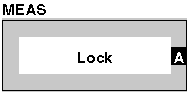The MEAS Lock button returns a 1.0 if the loop is locked, and a 0.0 if the loop is unlocked.

The MEAS Lock button will only function if 'Pll Mode' is set to 'Lock'. Then the parametric measure's 'Measure Mode' (from the Tune Measure panel) determines whether the MEAS Frequency button or the MEAS Lock button will function. If 'Measure Mode' is set to 'None', then the MEAS Lock button will function. If 'Measure Mode' is set to anything except 'None', then the MEAS Frequency button will function.

vs Time Measurements Panel, State Buttons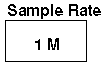The Sample Rate button defines the rate for the measurement vs. time buttons. It sets the rate at which measurements are taken. Together with the 'Samples' button, the 'Sample Rate' button defines the total measurement time for the 'vs. time' buttons.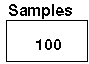The Samples button is used by the measurement vs. time buttons and the VCO measurement buttons. It sets the number of measurements taken.

For 'vs Time' measurements the maximum is 1024 and, together with the 'Sample Rate' button, the 'Samples' button defines the total measurement time for the 'vs Time' buttons.

For the VCO measurement buttons, the 'Samples' button defines the number of measurements for the DSP, with a maximum of 3000.

vs Time Measurements Panel, Measurement Buttons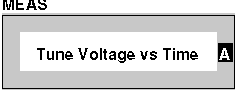The Tune Voltage vs Time button measures the voltage on the 'Charge Pump Out' line. This button is operational when the 'Measure Mode' button (in the 'Tune Measure' panel) is set to anything except 'None'.

This performs the same measurement as the 'Tune Voltage' button, except this button takes multiple 'Samples', timed by the 'Sample Rate' button.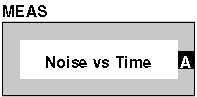The Noise vs Time button measures the raw AC (noise) output of the discriminator, through a DC block to eliminate DC. Used for raw FM/noise measurements, for debug or for uncorrected measurements. Returns a complex voltage vs. time.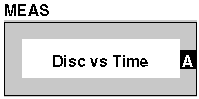The Disc vs Time button measures the DC (carrier) output of the discriminator through a low pass filter to eliminate AC. Used to get carrier frequency information. Returns a complex voltage vs. time.

VCO Measurements Panel, State Buttons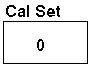The Cal Set button is used primarily for debug. It defines a rotation value for the I and Q, so that the DSP will work on rotated information. It must be an LVSet where the local var has a collection of the cal data.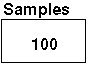The Samples button is used by the measurement vs. time buttons and the VCO measurement buttons. It sets the number of measurements taken.

For 'vs Time' measurements the maximum is 1024 and, together with the 'Sample Rate' button, the 'Samples' button defines the total measurement time for the 'vs Time' buttons.

For the VCO measurement buttons, the 'Samples' button defines the number of measurements for the DSP, with a maximum of 3000.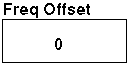The Freq Offset button sets the offset from the carrier, in Hz, for the VCO Measurement buttons.

VCO Measurements Panel, Measurement Buttons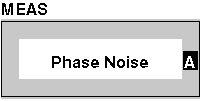The Phase Noise button measures the noise power at the offset frequency, assuming a 1 Hz bandwidth. The ratio is returned as a power ratio per Hz, and can be converted to dBc/Hz with a 'log' calc button.

It assumes noise and gives the ratio to the carrier per Hz. The ratio is one side of the carrier, the same as seen on a spectrum analyzer

The Phase Noise button is used for noise, whereas the Sideband button is used for single tones.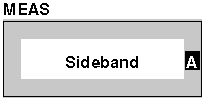The Sideband button measures the power, at the offset frequency, and returns a ratio relative to the carrier. The ratio is returned as a power ratio, and can be converted to dBc with a 'log' calc button.

It assumes an FM tone sideband and gives the ratio to the carrier. The ratio is B/2, one side of the carrier, the same as seen on a spectrum analyzer

The Sideband button is used for single tones, whereas the Phase Noise button is used for noise.

Tune Measure Panel, State Buttons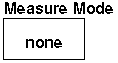The Measure Mode button selects the mode used for driving/measuring the 'Charge Pump Out' line.

If 'Pll Mode' is set to 'Lock', this button also determines whether the 'Measure Frequency' or 'Measure Lock' buttons are operational. See those buttons for further information.

None: The parametric measure does not drive the Charge Pump Out line, freeing the line for use by the other functions.

Meas V: Voltmeter mode. The parametric measure will measure the voltage on the line, without forcing anything on the line.
Force V: Forces a voltage on the line, set by the 'Measure V Force' button. The voltage or current on the line can be measured.
Force I: Forces a current on the line, set by the 'Measure I Force' button. The voltage or current on the line can be measured.
Leakage: Forces a voltage on the line, set by the 'Measure V Force' button. The voltage on the line can be measured. The current on the line can be measured using high resolution, low current circuitry with pA resolution and 50 uA maximum current.
Leak Cal: Not used for normal operation. Used when calibrating the TIM.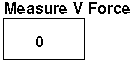The Measure V Force button sets the voltage when in the 'Force V' and 'Leakage' modes. This button has no effect in other modes. Range is -2 to +25V.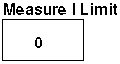The Measure I Limit button sets the maximum drive current when forcing voltage. If the current exceeds this value, the parametric measure will switch into current limit mode, and keep the current at this value.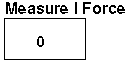The Measure I Force button sets the current when in the 'Force I' mode. This button has no effect in other modes. Range is ±2 mA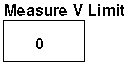The Measure V Limit button sets the maximum voltage when forcing current. If the voltage exceeds this value, the parametric measure will switch into voltage limit mode, and keep the voltage at this value.

Tune Measure Panel, Measurement Buttons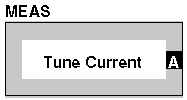The Tune Voltage button measures the voltage on the 'Charge Pump Out' line. This button is operational when the 'Measure Mode' button is set to anything except 'None'.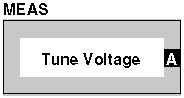The Tune Current button measures the current that the parametric measure is supplying to the 'Charge Pump Out' line. This button is operational when the Measure Mode button is set to anything except 'None', however it only measures the current supplied by the parametric measure. If other functions are forcing current onto the Charge Pump Out line, this button will not measure those currents.

Range is:

All modes except leakage: ±2 mA if 'Measure I Limit' is >500uA
±200 uA if 'Measure I Limit' is 500uA
Leakage mode: ±50 uA

https://roos.com/docs/RBES-8RJ62U
ROOS INSTRUMENTS CONFIDENTIAL AND PROPRIETARY